Home > CC4 > Chapter A > Lesson A.1.6 > ProblemA-63

A-63.

Evaluate the expressions below for the given values. Homework Help ✎

1. 6m + 2n2 for m = 7 and n = 3

2.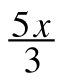− 2 for x = −18

3. (6x)2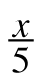for x = 10

4. (k − 3)(k + 2) for k = 1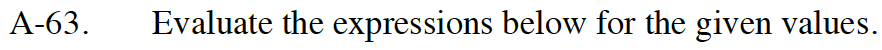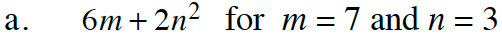6(7) + 2(3)²

Remember, the exponent only applies to the 3.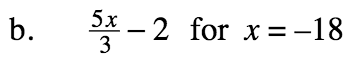You can simplify the fraction before multiplying 5 times x.

−32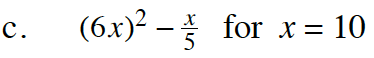Follow the order of operations.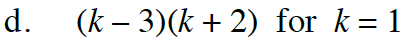−6